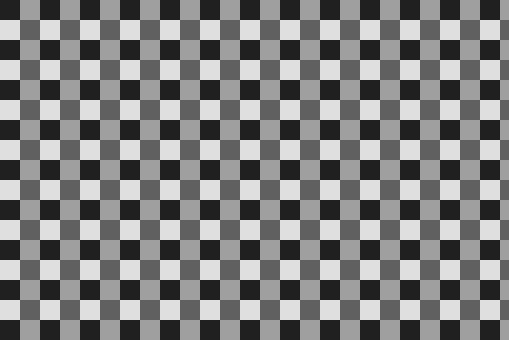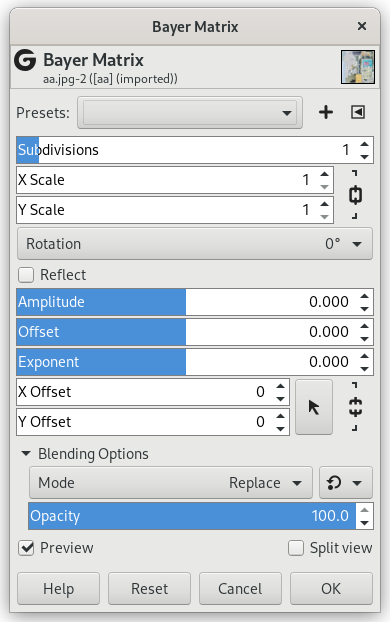### 14.11. Bayer Matrix

#### 14.11.1. Overview

Figure 17.344. Example for the Bayer Matrix filterBayer Matrix filter applied with Scale X, Y = 20

A grand-sounding name for a simple filter. Bayer matrix is related to ordered dithering, which is an image dithering algorithm used to display a continuous image on a display of smaller color depth. The algorithm reduces the number of colors by applying a threshold map known as Bayer matrix (Wikipedia). The present filter uses the Bayer matrix only to create a pattern.

#### 14.11.2. Starting filter

You can find this filter in the image menu through FiltersRenderPatternBayer Matrix…

#### 14.11.3. Options

Figure 17.345. Bayer Matrix filter optionsPresets, Preview, Split view
NoteThese options are described in Section 2, “Common Features”.
Subdivision

TODO

X Scale, Y Scale

Horizontal, Vertical pattern size.

Rotation

TODO

Reflect

Reflect the pattern horizontally.

Amplitude

TODO

Offset

TODO

Exponent

TODO

X, Y Offset

TODO